# Neural Networks Part 2: Building Neural Networks & Understanding Gradient Descent.

From the previous article , we learnt how a single neuron or perceptron works by taking the dot product of input vectors and weights,adding bias and then applying non-linear activation function to produce output.Now let’s take that information and see how these neurons build up to a neural network.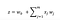Single Output Neuron

Now z=W0+∑ xj*wj denotes the dot product of input vectors and weights and our final output y is just activation function applied on z.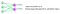Multi Output neuron

Now,if we want a multi output neural network(from the diagram above) ,we can simply add one of these perceptrons & we have two outputs with a different set of weights and inputs.Since all the inputs are densely connected to all the outputs,these layers are also called as Dense layers.To implement this layer, we can use many libraries such keras,tensorflow,pytorch,etc. Here it shows the tensorflow implementation of this 2 perceptron network where units =2 indicate we have two outputs in this layer.We can customize this layer by adding activation function,bias constraint etc.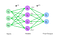Single Hidden Layer Neural Network

Now,let’s take a step further and let’s understand how a single layer neural network works where we have a single hidden layer which feeds into the output layer.

We call this a hidden layer because unlike our input and output layer which we can see or observe them.Our hidden layers are not directly observable,we can probe inside the network and see them using tools such as Netron but we can’t enforce it as these are learned .

Since there is a transformation between the input layer and hidden layer and hidden layer and output layer,these two transformations will have their own set of matrices W1 and W2(refer diagram above)where W1 corresponds to the weights of the first hidden layer.

So now if we look at a single hidden neuron z2 which takes the input and weights as z2=W1,2+W2,2+….+Wm,2.Now we can represent this in the form of code using tensorflow.

In code ,we can define these two dense layers ,the 1st one being our hidden layer with n outputs (you can decide any n value) and 2nd one being our output layer with 2 outputs and we can join them or aggreagate them together into this wrapper called as TF Sequential model.

Sequential models are just a concept of composing neural networks together using a sequence of layers so when you have a sequential processing system or data throughout the network(for eg. Processing a sentence through a model),you can use sequential model and define your layers as a sequence.

Now if we want to create a deep neural network,the idea is basically the same thing,you just keep stacking more of these layers and to create more of a hierarchical model where the final output is computed by going deeper and deeper into the network.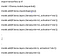Deep Neural Networks

The code is similar again we have a TF-Sequential model and inside that we have to define the layers we want to use and stack on top of each other.

So now that we have an idea of what is an neural network is and how a single neuron works ,let’s apply this neural network to a real life problem to train the model to accomplish some task . Suppose we take the following example of a two input/feature model where we define the features as No. of lectures you attend and How much you scored on your last exam?Let’s take all the data from previous years and plot it (diagram below). Green points represents the students that have passed the class in the past and red points represents the students who have failed the class .

We can plot this data in a 2-dimensional diagram like this and we can also plot YOU .Suppose you have attended 5 lectures and scored 4 marks (for the sake of this example out of 8). And the question is whether you are gonna pass the class?Given everyone around you and how they have done in the past,how are you gonna do?

We have 2 inputs,a single hidden layer consisting of 3 hidden units/neurons in that layer and an output.We see that the final output probability when we feed in those inputs of and 5 is 0.1 or 10%. Actual Result which we were expecting was 1 so YOU did pass the class but why was our network wrong in this example?Because we never told this network anything! We just initialized the weights ,in fact it has no idea what it means to pass a class and no idea what these inputs mean ,about how many lectures you attended and how many marks you scored.It is just seeing random numbers and it has no understanding of how many other people have scored so far .So what we have to do to this network first is train it,we have to teach it how to perform this task.Until we don’t teach it,it is just like a baby that doesn’t know anything which has just entered the world.So how do we teach it?

So first we have to tell the network where it is wrong,we have to quantify what it’s called a loss or error.And to do that we actually just take our prediction or what the network predicts and compare it to the true answer or the actual prediction which is 1 in this case.If there is a big difference between the actual and predicted value,we can tell the network,hey you made a big error here so you should try to fix your answer to move closer towards the actual answer.Now you can imagine you don’t have just 1 student but many students so the total loss which is also called as the Empirical loss, cost function,objective function is just the average of all those individual student losses so the individual loss is the loss(difference) between your prediction and actual value.That’s telling you how wrong a single example is and the final or total loss is the average of all those individual student losses.So if we look at the case of binary classification problem, which is this same example in this case where we are asking a question whether I will pass the class YES or NO?Binary Classification .We can use something called as the Binary Cross Entropy loss .

Cross Entropy loss can be used with models which output a probability between 0 and 1 .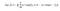Binary Cross Entropy

So instead of a classification problem lets assume this was a regression problem where instead of predicting whether you will pass the class or not ,you want to predict the final grade you are going to get.So now its not a YES?NO answer problem anymore but instead its whats the grade I’m going to get ,it’s a full range of numbers that are possible now.We might want to use a different type of loss for this different type of problem and we can do whats called a mean squared error loss.

Mean squared error loss can be used with regression models to output continuous real numbers.In this we take the actual grade and the predicted grade and subtract them ,take the mean squared error and say that’s the loss your network should try to minimize.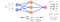Mean Squared Error

The goal now is to optimize his loss or to achieve the lowest loss.Our cost function or objective function W* is just the aggreagation and collection of individual w’s(losses )from all your weights.Its a very complicated problem but remember our loss function is just a function which we term in those weights.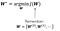Cost function is collection of individual losses

So if we plot this in terms of a 2-dimensional weight problem.One of the weights W0 on X-axis & the other W1 on Y axis and Z –axis represents our loss.So for any value of w ,we can see what the loss would be at that point.And what we want to do is we want to find out a place in this landscape that we get the minimum loss.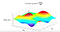Pick a point and compute gradient till we reach global minimumn

So what we can do is pick a random value on the landscape J(w0,w1) and then from this random place try to understand how the landscape is changing,whats the slope of the landscape.

We can take the gradient of the loss with respect to each of the landscapes.If we know which way is up we take a step in the direction that’s down.So we know which way is up so we reverse the sign,so we start heading downhill,we can moving downwards and move towards our lowest point.And we just keep repeating this process over and over again till we have converged to a global minimum.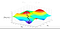Keep Calculating gradient till we reach the global minimun

Now we can summarize this algorithm which is called as gradient descent in which you are taking a gradient and descending down that landscape by starting to initialize our weights randomly ,compute the gradient with respect to all your weights,then we update our weights in the opposite direction of that gradient and take a small step which is called as learning rate (η).

Learning rate (η) is a scalar number which determines how much of a step you want to take in each direction.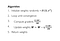Gradient Descent Algorithm

So how do we calculate that gradient? Given our loss,weights how do we know which is a good place to move given all this information and that can be done using a process called backpropagation.

In conclusion,remember that neural networks are these layers of neurons stacked together to create more of a hierarchical model where we train the network by reducing its loss or teaching the network where it wrong.And we try to reduce the loss by calculating the gradient(slope) till we reach the global minimum .

So this was all about neural networks are formed.Next article will demonstrate how we can calculate gradient and how network learns through backpropagation.

Happy Learning

## Analytics Vidhya

Analytics Vidhya is a community of Analytics and Data…

Written by

## Shweta Kadam

Software Engineer and always a Learner! https://shwetarkadam.github.io/portfolio

## Analytics Vidhya

Analytics Vidhya is a community of Analytics and Data Science professionals. We are building the next-gen data science ecosystem https://www.analyticsvidhya.com

Written by

## Shweta Kadam

Software Engineer and always a Learner! https://shwetarkadam.github.io/portfolio## Analytics Vidhya

Analytics Vidhya is a community of Analytics and Data Science professionals. We are building the next-gen data science ecosystem https://www.analyticsvidhya.com

## More From Medium

Medium is an open platform where 170 million readers come to find insightful and dynamic thinking. Here, expert and undiscovered voices alike dive into the heart of any topic and bring new ideas to the surface. Learn more

Follow the writers, publications, and topics that matter to you, and you’ll see them on your homepage and in your inbox. Explore

If you have a story to tell, knowledge to share, or a perspective to offer — welcome home. It’s easy and free to post your thinking on any topic. Write on Medium